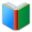# 4th Grade Cards - Math

Words for students of 4th Grade, the page is for math words (List 1), which are essential vocabulary for 4th Grade academic classes.
 Free Online Vocabulary TestK12, SAT, GRE, IELTS, TOEFL
 Actions upon current list

 All lists of current grdae
Spelling Words:

Arts Words:

Social Words:

Math Words:

Science Words:

Literature Words:Alice's AdventuresThe Fir TreeThe Snow QueenThe Goose GirlSnowdropAshputtelLily and the LionThe RavenTom Thumb
 4th Grade Cards - Math (click the card to toggle it.)

(You can also click each card to show or hide its content.)
plane surface, as of the floor of a room; open space in a building; the enclosed space; extent; scope; range
a chart with bars whose lengths are proportional to quantities
numbered or proceeding by tens; based on ten; a proper fraction whose denominator is a power of 10
the divisor of a fraction
a special way in which multiplication is applied to addition of two or more numbers in which each term inside a set of parentheses can be multiplied by a factor outside the parentheses
one of five equal parts of a whole
one of a hundred equal parts of a whole; called also percent
a graph in which a series of connected straight lines connect points, each of which represents the value of a particular variable
the value below which 50% of the cases fall
prevailing style; manner; way of doing something; fashion or style
the dividend of a fraction
make or place something to another's side; of or relating to multiple operations at same time; not intersecting
outer boundary length; closed curve bounding a plane area
vertical; upright; intersecting at or forming right angles; extremely steep
percentage; quotient obtained when a part is divided by the whole; part considered in relation to the whole
a quarter of the circumference of a circle; any of the four areas into which a plane is divided by two orthogonal coordinate axes
limits within which something can be effective; variety of different things or activities
an integer or a fraction
image of something as reflected by mirror; remark expressing careful consideration
revolution; act of rotating as if on an axis
one of ten equal parts of a whole
change in form , appearance, nature, disposition, condition, or the like
transformation in which the origin of the coordinate system is moved to another position but the direction of each axis remains the same
one of twelve equal parts of a whole
 Quick Access Worksheet: